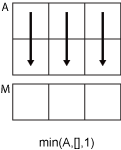min

说明

M = min(A) 返回数组的最小元素。

• 如果 A 是向量，则 min(A) 返回 A 的最小值。

• 如果 A 为矩阵，则 min(A) 是包含每一列的最小值的行向量。

• 如果 A 是多维数组，则 min(A) 沿大小不等于 1 的第一个数组维度计算，并将这些元素视为向量。此维度的大小将变为 1，而所有其他维度的大小保持不变。如果 A 是第一个维度为 0 的空数组，则 min(A) 返回与 A 大小相同的空数组。

M = min(A,[],dim) 返回维度 dim 上的最小元素。例如，如果 A 为矩阵，则 min(A,[],2) 是包含每一行的最小值的列向量。

M = min(A,[],nanflag) 指定在计算中包括还是忽略 NaN 值。例如，min(A,[],'includenan') 包括 A 中的所有 NaN 值，而 min(A,[],'omitnan') 忽略这些值。

M = min(A,[],dim,nanflag) 还指定使用 nanflag 选项时的运算维度。

[M,I] = min(___) 在上述语法基础上返回 A 中最小值在运算维度上的索引。

M = min(A,[],'all') 查找 A 的所有元素的最小值。此语法适用于 MATLAB® R2018b 及更高版本。

M = min(A,[],vecdim) 计算向量 vecdim 所指定的维度上的最小值。例如，如果 A 是矩阵，则 min(A,[],[1 2]) 计算 A 中所有元素的最小值，因为矩阵的每个元素都包含在由维度 1 和 2 定义的数组切片中。

M = min(A,[],'all',nanflag) 计算在使用 nanflag 选项时 A 的所有元素的最小值。

M = min(A,[],vecdim,nanflag) 指定在使用 nanflag 选项时要运算的多个维度。

[M,I] = min(A,[],___,'linear') 返回 A 中最小值在 A 中的线性索引。

C = min(A,B) 返回从 AB 中提取的最小元素的数组。

C = min(A,B,nanflag) 还指定如何处理 NaN 值。

示例

A = [23 42 37 15 52];
M = min(A)
M = 15

A = [-2+2i 4+i -1-3i];
min(A)
ans = -2.0000 + 2.0000i

A = [2 8 4; 7 3 9]
A = 2×3

2     8     4
7     3     9

M = min(A)
M = 1×3

2     3     4

A = [1.7 1.2 1.5; 1.3 1.6 1.99]
A = 2×3

1.7000    1.2000    1.5000
1.3000    1.6000    1.9900

M = min(A,[],2)
M = 2×1

1.2000
1.3000

A = [1.77 -0.005 3.98 -2.95 NaN 0.34 NaN 0.19];
M = min(A,[],'omitnan')
M = -2.9500

M = min(A,[],'includenan')
M = NaN

A = [1 9 -2; 8 4 -5]
A = 2×3

1     9    -2
8     4    -5

[M,I] = min(A)
M = 1×3

1     4    -5

I = 1×3

1     2     2

A(:,:,1) = [2 4; -2 1];
A(:,:,2) = [9 13; -5 7];
A(:,:,3) = [4 4; 8 -3];
M1 = min(A,[],[1 2])
M1 =
M1(:,:,1) =

-2

M1(:,:,2) =

-5

M1(:,:,3) =

-3

M2 = min(A,[],[1 2 3])
M2 = -5
Mall = min(A,[],'all')
Mall = -5

A = [1 2 3; 4 5 6]
A = 2×3

1     2     3
4     5     6

[M,I] = min(A,[],2,'linear')
M = 2×1

1
4

I = 2×1

1
2

minvals = A(I)
minvals = 2×1

1
4

A = [1 7 3; 6 2 9]
A = 2×3

1     7     3
6     2     9

B = 5;
C = min(A,B)
C = 2×3

1     5     3
5     2     5

输入参数

• 如果 A 是复矩阵，则 min(A) 返回幅值最小的复数。如果幅值相等，则 min(A) 返回具有最小幅值和最小相位角的值。

• 如果 A 是标量，则 min(A) 返回 A

• 如果 A 为 0×0 空数组，则 min(A) 也是空数组。

• 如果 dim = 1，则 min(A,[],1) 返回包含每列中的最小元素的行向量。• 如果 dim = 2，则 min(A,[],2) 返回包含每行中的最小元素的列向量。• AB 的数据类型必须相同，除非其中一个是 double。如果是那样，另一个数组的数据类型可以是 singleduration 或任何整数类型。

• 如果 AB 均为有序 categorical 数组，则必须具有顺序相同的同一类别集。

NaN 条件，指定为下列值之一：

• 'omitnan' - 忽略输入中的所有 NaN 值。如果所有元素均为 NaN，则 min 返回第一个元素。

• 'includenan' - 在计算输入中包括 NaN 值。

输出参数

AB 中的最小元素，以标量、向量、矩阵或多维数组的形式返回。C 的大小由 AB 的维度的隐式扩展决定。有关详细信息，请参阅基本运算的兼容数组大小

C 的数据类型取决于 AB 的数据类型：

• 如果 AB 是相同的数据类型，则 CAB 的数据类型匹配。

• 如果 ABsingle，则 Csingle

• 如果 AB 为整数数据类型且另一个为标量 double，则 C 采用整数数据类型。